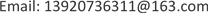1. 引言

2. 基于飞机垂直加速度的EDR估计2.1. 基本思想

ε = B σ T 3 V − 1 (1)

2.2. 飞机响应因子

B 是特定飞机的响应因子，取决于飞机特性(机翼面积，飞机质量等)和飞行条件(真空速，飞行高度等)  。

H r g ( ω ) = z ¨ w g 0 = − ω 2 − 1 2 ρ V S W a − ω 2 m + i ω 1 2 ρ V S W a (2)

B = 1 / [ 0.7 3 / 2 I 3 / 2 ] (3)

I = ∫ | H r g ( ω ) | 2 ω − 5 / 3 d ω (4)

d z d t = v z (5)

d v z d t = a z (6)

Categorization of turbulence intensit
EDR的取值范围湍流强度
EDR ≤ 0.15无湍流
0.15 < EDR ≤ 0.3轻度湍流
0.3 < EDR ≤ 0.55中度湍流
0.55 < EDR ≤ 0.8重度湍流

3. CAT对飞机的影响量化3.1. 基于垂直载荷因子的晴空湍流检测方法的基本思想

σ ^ Δ n = σ Δ n u n i t σ w [ M ¯ 2 ( x ) ] 0.5 σ v 2 ( r ) σ (7)

3.2. 垂直载荷因子与EDR的关系

4. 验证和分析

5. 结论